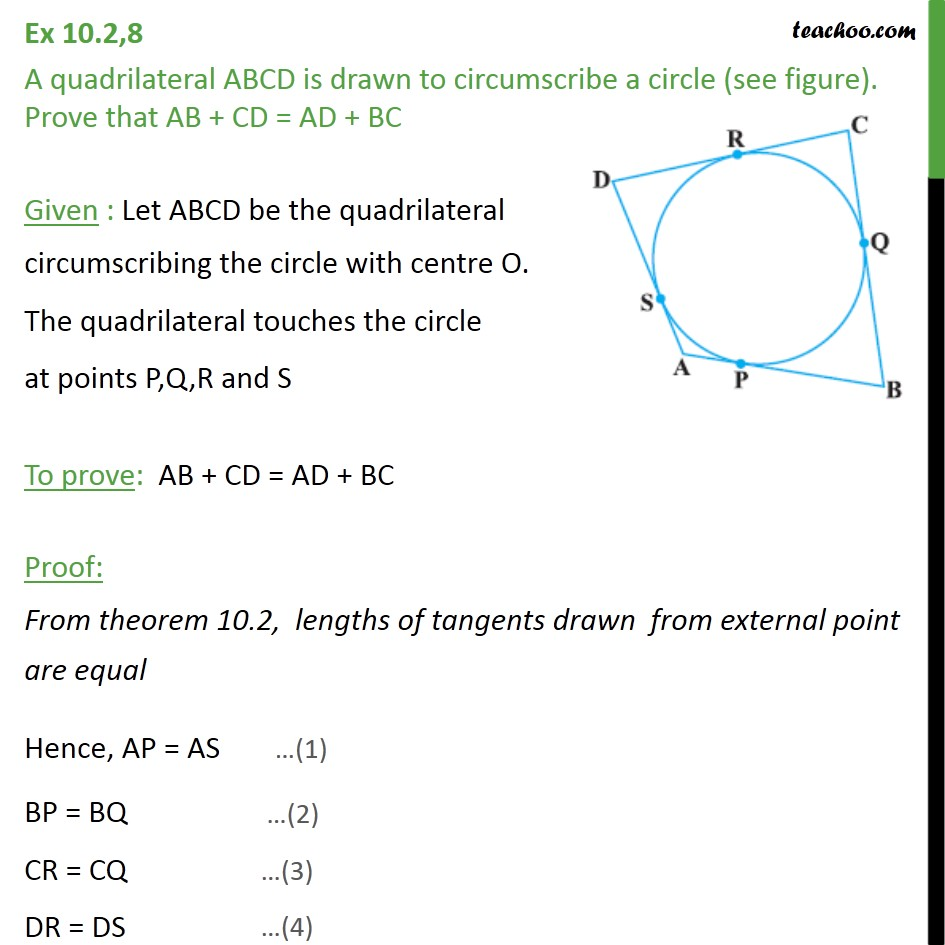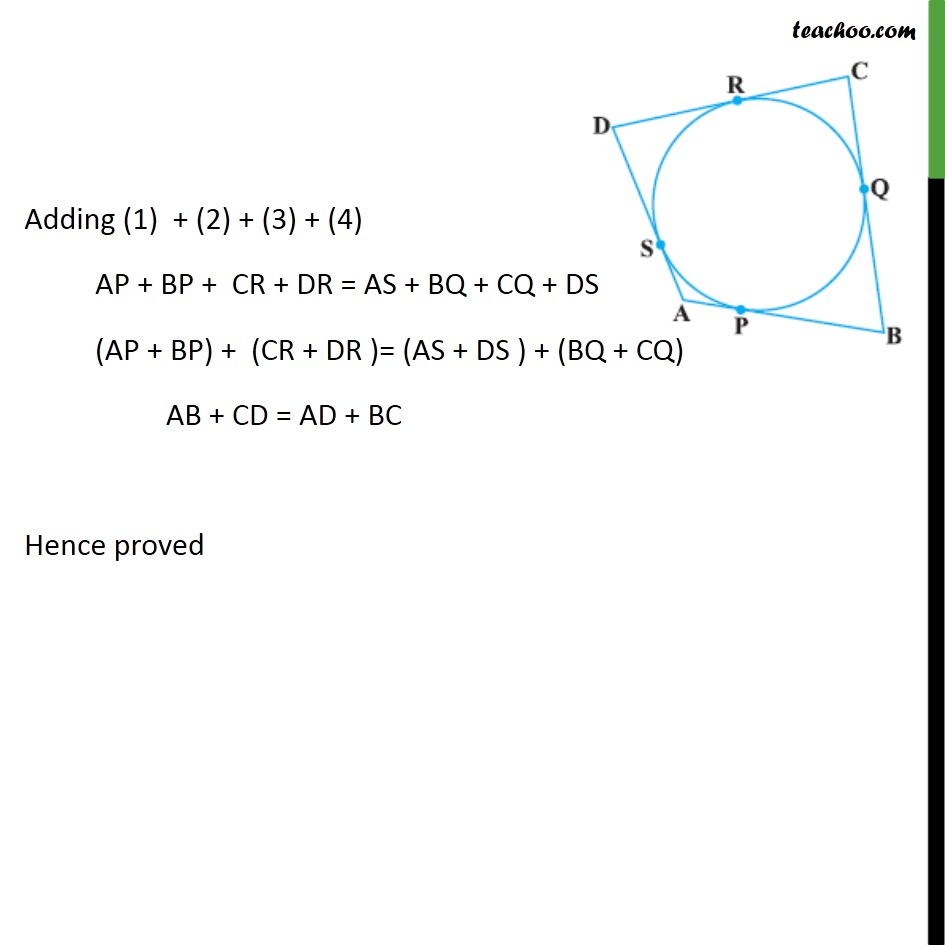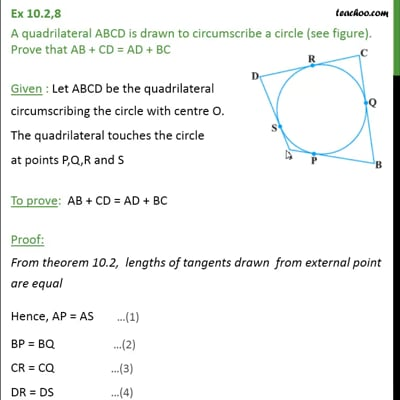Ex 10.2

Chapter 10 Class 10 Circles
Serial order wiseThis video is only available for Teachoo black users

Learn in your speed, with individual attention - Teachoo Maths 1-on-1 Class

### Transcript

Ex 10.2,8 A quadrilateral ABCD is drawn to circumscribe a circle (see figure). Prove that AB + CD = AD + BC Given : Let ABCD be the quadrilateral circumscribing the circle with centre O. The quadrilateral touches the circle at points P,Q,R and S To prove: AB + CD = AD + BC Proof: From theorem 10.2, lengths of tangents drawn from external point are equal Hence, AP = AS BP = BQ CR = CQ DR = DS Adding (1) + (2) + (3) + (4) AP + BP + CR + DR = AS + BQ + CQ + DS (AP + BP) + (CR + DR )= (AS + DS ) + (BQ + CQ) AB + CD = AD + BC Hence proved